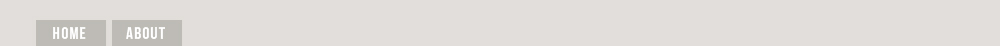## Dec 19, 2016

### Turning Big Data Into Manageable Data

Big data is just getting so darned big that enterprises risk the inability to effectively, productively and affordably utilize it. The resulting solutions required will be innovations in management and analytical process in order to optimize the cost, time- benefit continuum. JL

Kevin Murnane reports in Forbes:

Data analysis tools such as principal-component analysis are commonly used to reduce the number of variables in a dataset. Unfortunately, using these tools on massive big data sets is often too time consuming to be practical. Reducing big-data into a coreset preserves the important mathematical relationships that are present in the big data and is small enough to be used effectively by a variety of data analysis techniques.
Big data is ubiquitous because it can provide valuable insight that is unavailable without it. Analyzing big data sets can pose problems, however. For starters, big data is big, sometimes too big to be handled effectively by commonly used analysis tools. Researchers at MIT’s Computer Science and Artificial Intelligence Laboratory and the University of Haifa in Israel have developed a solution to this problem that turns big data into manageable data.
Data analysis tools such as low-rank approximation, singular-value decomposition, principal-component analysis, and nonnegative matrix factorization are commonly used to reduce the number of variables in a dataset. Unfortunately, using these tools on massive big data sets is often too time consuming to be practical.
A typical solution for this problem involves finding a coreset for the big data set. A coreset is a subset of the big data that preserves the big data’s most important mathematical relationships. Data analysis tools can work more effectively with the coreset because it’s smaller. Finding a coreset can be a problem if you want to compare two or more data analyses because each of the analysis tools has its own unique method for extracting a coreset from the big data. Comparing results across analyses involves comparing results derived from different coresets which is not ideal. The research team solved this problem by developing a general method for extracting a coreset that can be used by a large group of commonly used data analysis tools.
Suppose you wanted to identify the topics that appear most often in an immense text database like Wikipedia. Low-rank approximation is an algorithm that will do the job but the Wikipedia database is so big that low-rank approximation will take too long to finish the task.
How big is the Wikipedia database? Imagine a matrix or table that has a row for each article in Wikipedia and a column for each word that appears in Wikipedia. This matrix would have 1.4 million rows for the articles and 4.4 million columns for the words. That’s a table with about 6.2 trillion cells which is about 821 cells for every person on earth. Big data, indeed.
The researcher’s solution uses an advanced type of geometry to shrink this enormous data set into a coreset that is more manageable. Imagine a rectangle in two dimensions, length and width. Easy to do. Now add a third dimension, depth. It’s a box, also easy to imagine. Now add a fourth dimension, time. We call it space-time but it’s not so easy to imagine. Now add two or three more dimensions and imagine what it looks like. Good luck with that.

We can’t imagine what these multidimensional spaces look like but we can do geometry in them. In order to shrink the Wikipedia matrix the researchers used a multidimensional circle called a hypercircle that has 4.4 million dimensions, one for each word that appears in Wikipedia. Each of the 1.4 million articles in Wikipedia is represented as a unique point on this hypercircle.
How did the researcher shrink the hypercircle into something more manageable? Each of the 4.4 million words in Wikipedia is represented by a variable and each of the articles in Wikipedia is represented by its own unique set of values for these 4.4 million variables. The researcher’s hypercircle technique involves taking one article at a time and finding the average of a small subset of its 4.4 million variables, say 50 of them. The average that best preserves the mathematical relationships among the variables can be found by calculating the center of the much smaller 50-dimension hypercircle that represents the 50 variables or words. The average is then entered as one of the data points in the coreset. This process is repeated for the remaining variables (words) in each article and for each of the 1.4 million articles.
Reducing the big-data Wikipedia matrix into a coreset using this method takes a lot of individual calculations but each calculation can be carried out very quickly because it only involves 50 variables. The result is a coreset that preserves the important mathematical relationships that are present in the big data and is small enough to be used effectively by a variety of data analysis techniques.

The real beauty of the hypercircle technique lies in this variety. The technique creates a coreset that can be used by many of the data analysis tools that are often applied in computer vision, natural language processing, neuroscience, weather prediction, recommendation systems and more. You might even think of the hypercircle as the one ring that rules them all.